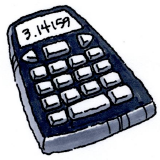### Home > AC > Chapter 8 > Lesson 8.2.4 > Problem8-80

8-80.

Calculate the value of each expression below using a scientific calculator.1.  $\large{\frac{-10+\sqrt{25}}{ 5}}$

• Calculate the square root, then simplify the rest of the expression.

• $\frac{-10+5}{5}$

1.  $\large{\frac{8+\sqrt{40}}{ 3\cdot3}}$

Calculate the square root and everything in the denominator before simplifying the rest of the expression.

1.  $\large{\frac{8+\sqrt{3^2+2\cdot3+1}}{-4}}$

Calculate everything inside the square root before simplifying.